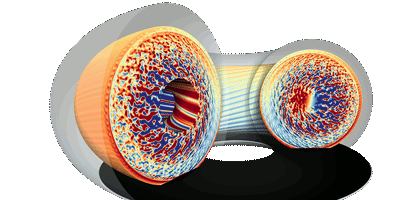# Gysela-X++

The Gysela-X++ code models turbulence in tokamak plasmas.The 5D GYSELA-X (for GYrokinetic SEmi-LAgrangian) code1 is developed at CEA/IRFM for 20 years through national and international collaborations with a strong interaction between physicists, mathematicians and computer scientists. GYSELA-X is a global full-f nonlinear gyrokinetic code that simulates electrostatic plasma turbulence and transport in the core of Tokamak devices. It evolves the complete (so-called “full-f”) 5-dimensional (3 space coordinates, 2 velocity coordinates) guiding-centre distribution function in the electrostatic limit in a full portion of torus (so-called “global”). Versatile sources of heat, momentum, particles and vorticity are commonly used to achieve steady-state in long simulation runs. The time-evolution of this distribution function $f(r,\theta,\phi,v_\parallel,\mu)$ is governed by the gyro-averaged Vlasov equation, the so-called gyrokinetic equation, which is self-consistently coupled to the 3D quasi-neutrality equation (electrostatic and long wavelength limit of Maxwell’s equations). From the numerical point of view, GYSELA-X is based on a semi-Lagrangian scheme.

1. [Virginie Grandgirard, Jérémie Abiteboul, Julien Bigot, Thomas Cartier-Michaud, Nicolas Crouseilles, et al.. A 5D gyrokinetic full-f global semi-lagrangian code for flux-driven ion turbulence simulations. 2015.] ↩︎